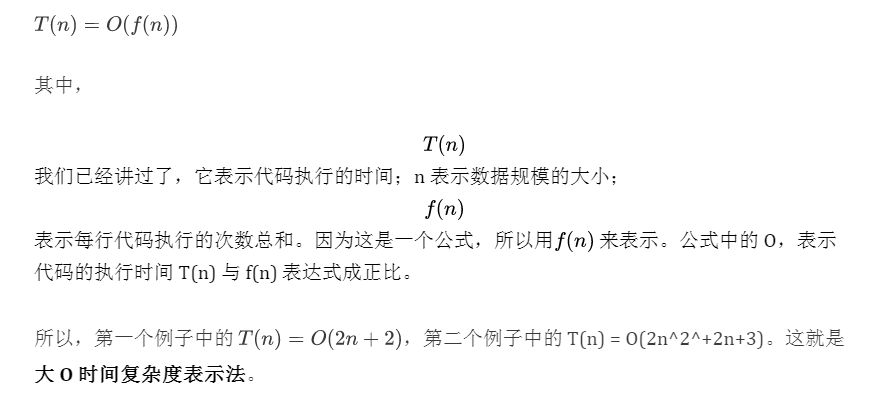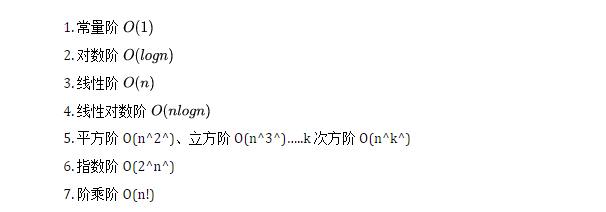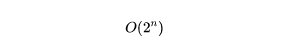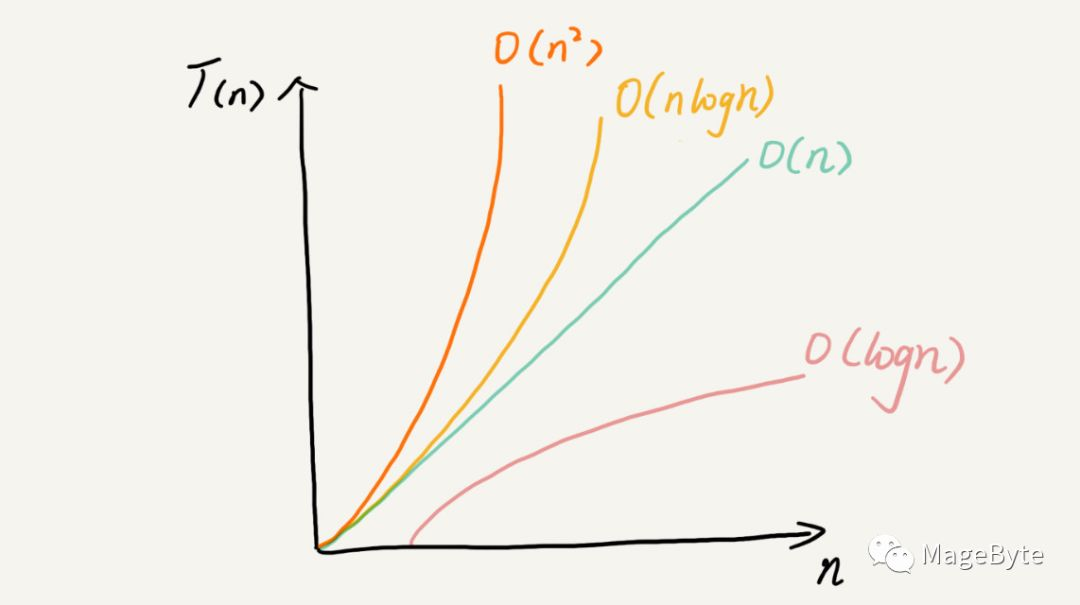# 2.时间复杂度与空间复杂度

0收藏1. 测试结果非常依赖测试环境

2.测试结果受数据规模的影响很大

``````int cal(int n) {
int sum = 0;
int i = 1;
for (; i <= n; ++i) {
sum = sum + i;
}
return sum;
}``````

``````int cal(int n) {
int sum = 0;
int i = 1;
int j = 1;
for (; i <= n; ++i) {
j = 1;
for (; j <= n; ++j) {
sum = sum +  i * j;
}
}
}``````1. 只关注循环执行次数最多的一段代码

``````int cal(int n) {
int sum = 0;
int i = 1;
for (; i <= n; ++i) {
sum = sum + i;
}
return sum;
}``````

2. 加法法则：总复杂度等于量级最大的那段代码的复杂度

``````int cal(int n) {
int sum_1 = 0;
int p = 1;
for (; p < 100; ++p) {
sum_1 = sum_1 + p;
}

int sum_2 = 0;
int q = 1;
for (; q < n; ++q) {
sum_2 = sum_2 + q;
}

int sum_3 = 0;
int i = 1;
int j = 1;
for (; i <= n; ++i) {
j = 1;
for (; j <= n; ++j) {
sum_3 = sum_3 +  i * j;
}
}

return sum_1 + sum_2 + sum_3;
}``````

● 第一段的时间复杂度是多少呢？这段代码循环执行了 100 次，所以是一个常量的执行时间，跟 n 的规模无关。
● 这里我要再强调一下，即便这段代码循环 10000 次、100000 次，只要是一个已知的数，跟 n 无关，照样也是常量级的执行时间。当 n 无限大的时候，就可以忽略。尽管对代码的执行时间会有很大影响，但是回到时间复杂度的概念来说，它表示的是一个算法执行效率与数据规模增长的变化趋势，所以不管常量的执行时间多大，我们都可以忽略掉。因为它本身对增长趋势并没有影响。
● 那第二段代码和第三段代码的时间复杂度是多少呢？答案是 O(n) 和 O(n^2^)，你应该能容易就分析出来，我就不啰嗦了。

``````如果 T1(n)=O(f(n))，T2(n)=O(g(n))；那么 T(n)=T1(n)+T2(n)=max(O(f(n)), O(g(n))) =O(max(f(n), g(n)))
``````

3. 乘法法则：嵌套代码的复杂度等于嵌套内外代码复杂度的乘积``````int cal(int n) {
int ret = 0;
int i = 1;
for(x=1; i <= n; x++){
for(i = 1; i <= n; i++) {
j = i;
j++;
}
}
}``````1. O(1) 之一击必杀

``````int a = 1;
int b = 2;
int c = 3;``````

2. O(logn)、O(nlogn)

``````i=1;
while (i <= n)  {
i = i * 2;
}```````````` i=1;
while (i <= n)  {
i = i * 3;
}````````````for(m = 1; m < n; m++) {
i = 1;
while(i < n) {
i = i * 2;
}
}``````

3. O(m+n)、O(m*n)

``````int cal(int m, int n) {
int sum_1 = 0;
int i = 1;
for (; i < m; ++i) {
sum_1 = sum_1 + i;
}

int sum_2 = 0;
int j = 1;
for (; j < n; ++j) {
sum_2 = sum_2 + j;
}

return sum_1 + sum_2;
}``````

**4.线性阶O(n) **

``````for(i=1; i<=n; i++) {
j = i;
j++;
}``````

``````for(x=1; i <= n; x++){
for(i = 1; i <= n; i++) {
j = i;
j++;
}
}
``````

``````void print(int n) {
int i = 0;
int[] a = newint[n];
for (i; i <n; ++i) {
a[i] = i * i;
}
}``````

``````int i = 1;
int j = 2;
++i;
j++;
int m = i + j;``````

``````int[] m = newint[n]
for(i=1; i <= n; ++i) {
j = i;
j++;
}``````有人说，我们项目之前都会进行性能测试，再做代码的时间复杂度、空间复杂度分析，是不是多此一举呢？而且，每段代码都分析一下时间复杂度、空间复杂度，是不是很浪费时间呢？你怎么看待这个问题呢？

《数据结构与算法之美》社区精华内容